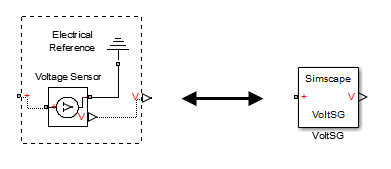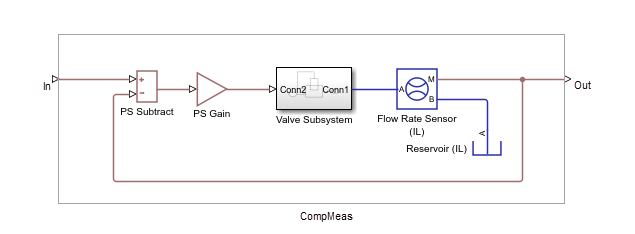## Specifying Component Connections

The structure section of a Simscape™ file is executed once during compilation. This section contains information on how the constituent components’ ports are connected to one another, as well as to the external inputs, outputs, and nodes of the top-level component.

The structure section begins with a `connections` keyword and is terminated by an `end` keyword. This `connections` block contains a set of `connect` constructs, which describe both the conserving connections (between `nodes`) and the physical signal connections (between the `inputs` and `outputs`).

In the following example, the custom component file includes the Foundation library Voltage Sensor and Electrical Reference blocks and specifies the following connections:

• Positive port of the voltage sensor to the external electrical conserving port + of the composite component

• Negative port of the voltage sensor to ground

• Physical signal output port of the voltage sensor to the external output of the composite component, located on the right side of the resulting block icon```component VoltSG nodes p = foundation.electrical.electrical; % + end outputs Out = { 0.0, 'V' }; % V:right end components(ExternalAccess=observe) VoltSensor = foundation.electrical.sensors.voltage; Grnd = foundation.electrical.elements.reference; end connections connect(p, VoltSensor.p); connect(Grnd.V, VoltSensor.n); connect(VoltSensor.V, Out); end end ```

In this example, the first two `connect` constructs specify conserving connections between electrical nodes. The third `connect` construct is a physical signal connection. Although these constructs look similar, their syntax rules are different.

### Conserving Connections

For conserving connections, the `connect` construct can have two or more arguments. For example, the connections in the following example

``` connections connect(R1.p, R2.n); connect(R1.p, R3.p); end```

can be replaced with

``` connections connect(R1.p, R2.n, R3.p); end```

The order of arguments does not matter. The only requirement is that the nodes being connected are all of the same type (that is, are all associated with the same domain).

In the following example, the composite component consists of three identical resistors connected in parallel:

```component ParResistors nodes p = foundation.electrical.electrical; n = foundation.electrical.electrical; end parameters p1 = {3 , 'Ohm'}; end components(ExternalAccess=observe) r1 = foundation.electrical.elements.resistor(R=p1); r2 = foundation.electrical.elements.resistor(R=p1); r3 = foundation.electrical.elements.resistor(R=p1); end connections connect(r1.p, r2.p, r3.p, p); connect(r1.n, r2.n, r3.n, n); end end ```

### Connections to Implicit Reference Node

The `*` symbol indicates connections to a reference node in `branch` statements. You can also use it to indicate connections to an implicit reference node within the structure section of a component:

```connections connect(A, *); end ```

The implicit reference node acts as a virtual grounding component. A node connected to an implicit reference has all its Across variables equal to 0.

The `*` symbol is not domain-specific, and the same structure section can contain connections to implicit reference in different domains:

```component abc nodes M = foundation.hydraulic.hydraulic; N = foundation.electrical.electrical; end connections connect(M,*); connect(N,*); end end```

However, multiple ports connected to an implicit reference within the same `connect` statement must all belong to the same domain:

```connections connect(a, b, *); end ```

The order of ports does not matter. This behavior is consistent with general connection rules for multiple conserving ports.

### Physical Signal Connections

Physical signal connections are directional, therefore the `connect` construct has the following format:

``` connect(s, d); ```

where `s` is the signal source port and `d` is the destination port.

There can be more than one destination port connected to the same source port:

``` connect(s, d1, d2); ```

The source and destination ports belong to the `inputs` or `outputs` member classes. The following table lists valid source and destination combinations.

SourceDestination
External input port of composite componentInput port of member component
Output port of member componentInput port of member component
Output port of member componentExternal output port of composite component

For example, consider the following block diagram.It represents a composite component `CompMeas`, which, in turn, contains a composite component `Valve Subsystem`, as well as several Foundation library blocks. The Simscape file of the composite component would specify the equivalent signal connections with the following constructs.

ConstructExplanation
`connect(In, subt.I1);`Connects port `In` to the input port `+` of the PS Subtract block. Illustrates connecting an input port of the composite component to an input port of a member component.
`connect(subt.O, gain.I);`Connects the output port of the PS Subtract block to the input port of the PS Gain block. Illustrates connecting an output port of a member component to an input port of another member component at the same level.
`connect(fl_rate.Q, subt.I2, Out);`Connects the output port `Q` of the Hydraulic Flow Rate Sensor block to the input port `-` of the PS Subtract block and to the output port `Out` of the composite component. Illustrates connecting a single source to multiple destinations, and also connecting an output port of a member component to an output port of the enclosing composite component.

Also notice that the output port of the PS Gain block is connected to the input port of the Valve Subsystem composite block (another member component at the same level). Valve Subsystem is a standalone composite component, and therefore if you connect the output port of the PS Gain block to an input port of one of the member components inside the Valve Subsystem, that would violate the causality of the physical signal connections (a destination port cannot be connected to multiple sources).

### Nonscalar Physical Signal Connections

Multidimensional physical signals can be useful for:

• Aggregating measurements at different spatial points, such as temperatures along a coil or a 2-D grid of elements

• Using 3-D body positions or velocities

• Using rotation matrices or quaternions in 3-D

• Using tensors

Simscape language supports nonscalar (vector-valued or matrix-valued) physical signals in `inputs` and `outputs` declarations. All signals in such vector or matrix should have the same units. For example, the following declaration

``` inputs I = {zeros(3), 'm/s'}; % :left end ```

initializes a component input as a 3-by-3 matrix of linear velocities.

When you connect input and output ports carrying nonscalar physical signals, you can use signal indexing and concatenation at the source, but not at the destination. Scalar expansion is not allowed.

The following table shows valid syntax examples, assuming subcomponent A with output signal port `A.o` is being connected to subcomponent B with input signal port `B.i`, and all sizes and units are compatible.

ConstructExplanation
`connect(A.o(1,2), B.i);`Source indexing, to connect to a scalar destination: take entry (1,2) of the output A.o and connect it to the input B.i.
`connect(A.o(1:2:5,2:3), B.i);`Index by rows and columns to specify a submatrix.
`connect(A.o(1:2:end,:), B.i);`Use colon notation to specify array boundaries (pass every other column of the output A.o to input B.i.
`connect([A1.o, A2.o], B.i);`Concatenate outputs A1.o and A2.o column-wise and pass the result to the input B.i.

You can use block parameter values for indexing inside a `connect` statement, for example:

```connect(a.o(value(param_name, '1'), 3), b.i);```

When you connect two physical signals, their units must be directly convertible. If one of the signals is declared as unitless (that is, with units of `'1'`) , then you can connect a signal with any base units to it. However, unit conversion is not supported in this case. For example, if `a.i` is a 2x1 unitless input port, then this statement is valid:

`connect([out1_in_meters, out2_in_seconds], a.i); `

If you connect signals with different scales of the same unit with a unitless input port, the compiler alerts you to the fact that unit conversion is ignored. For example, the following statement produces a warning at compile time:

`connect([out1_in_km, out2_in_mm], a.i); `